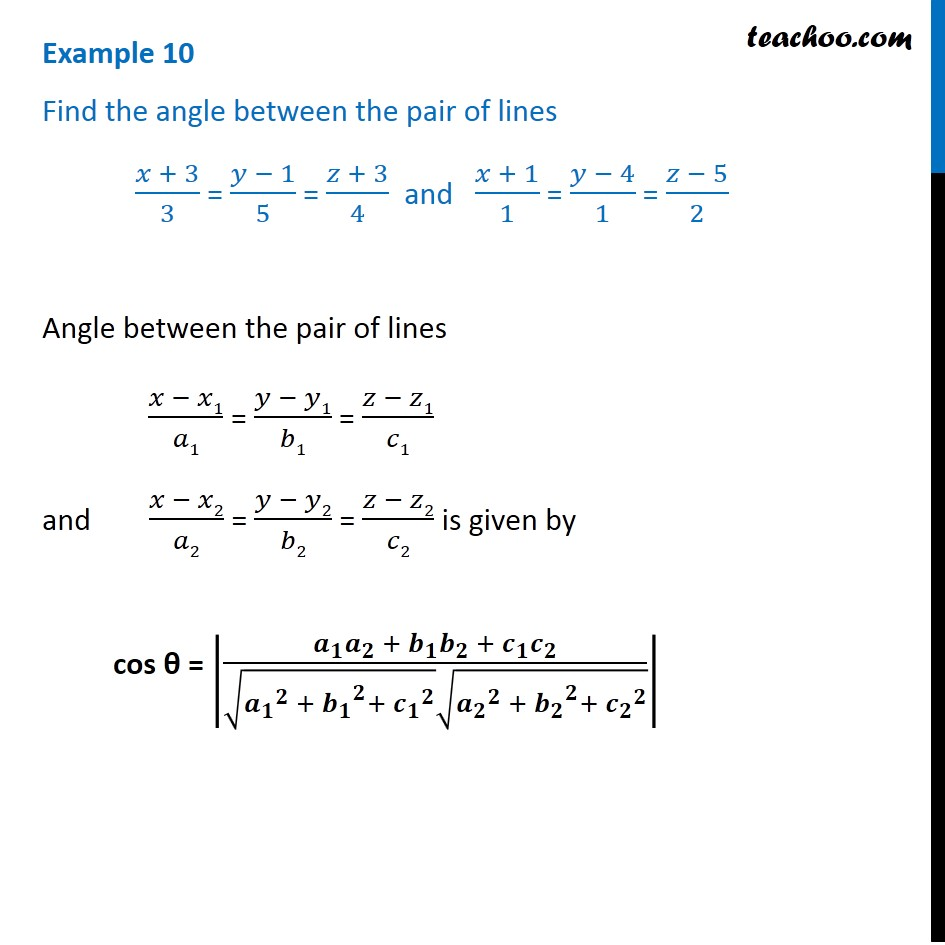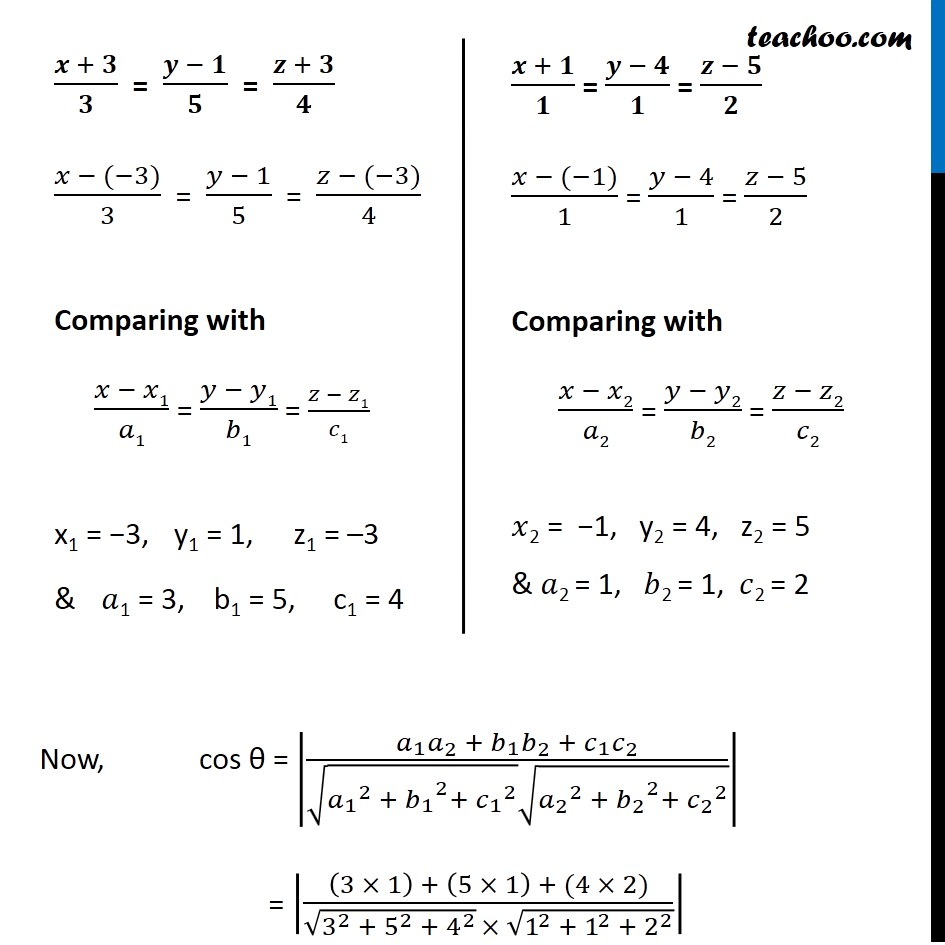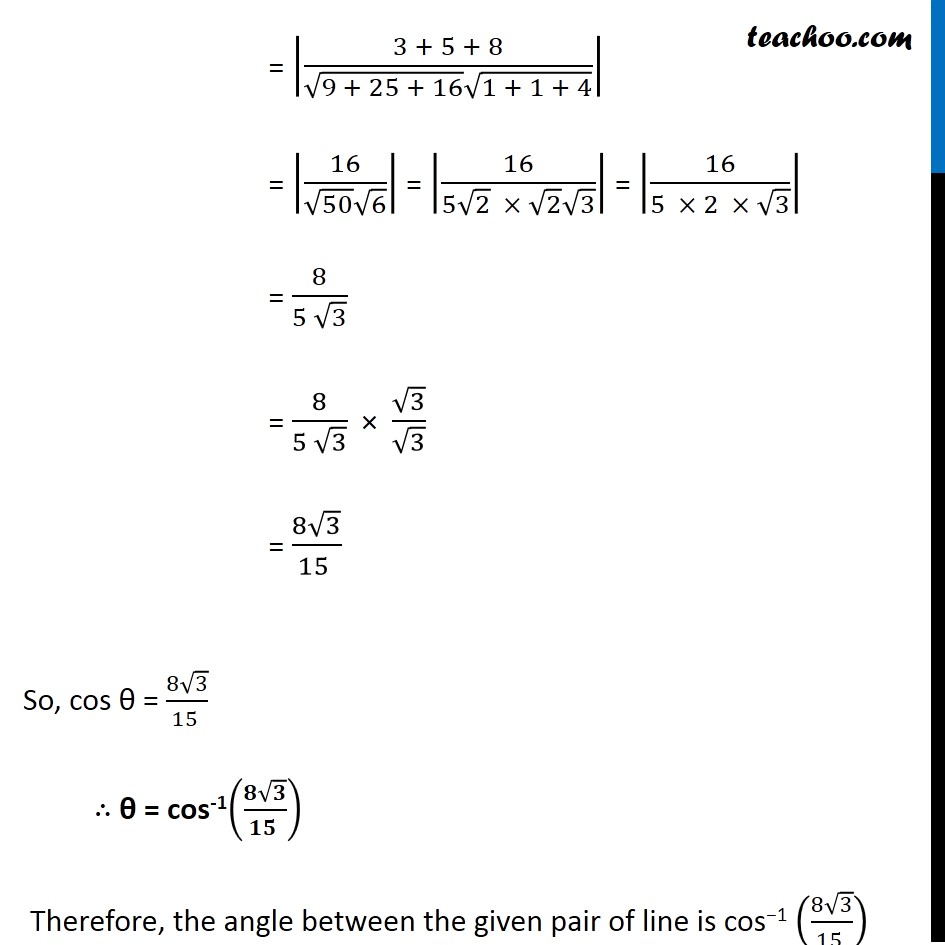Examples

Chapter 11 Class 12 Three Dimensional Geometry
Serial order wiseLearn in your speed, with individual attention - Teachoo Maths 1-on-1 Class

### Transcript

Example 8 Find the angle between the pair of lines (𝑥 + 3)/3 = (𝑦 − 1)/5 = (𝑧 + 3)/4 and (𝑥 + 1)/1 = (𝑦 − 4)/1 = (𝑧 − 5)/2Angle between the pair of lines (𝑥 − 𝑥1)/𝑎1 = (𝑦 − 𝑦1)/𝑏1 = (𝑧 − 𝑧1)/𝑐1 and (𝑥 − 𝑥2)/𝑎2 = (𝑦 − 𝑦2)/𝑏2 = (𝑧 − 𝑧2)/𝑐2 is given by cos θ = |(𝒂_𝟏 𝒂_𝟐 + 𝒃_𝟏 𝒃_𝟐 +〖 𝒄〗_𝟏 𝒄_𝟐)/(√(〖𝒂_𝟏〗^𝟐 + 〖𝒃_𝟏〗^𝟐+ 〖𝒄_𝟏〗^𝟐 ) √(〖𝒂_𝟐〗^𝟐 +〖〖 𝒃〗_𝟐〗^𝟐+ 〖𝒄_𝟐〗^𝟐 ))| (𝒙 + 𝟑)/𝟑 = (𝒚 − 𝟏)/𝟓 = (𝒛 + 𝟑)/𝟒 (𝑥 − (−3))/3 = (𝑦 − 1)/5 = (𝑧 − (−3))/4 Comparing with (𝑥 − 𝑥1)/𝑎1 = (𝑦 − 𝑦1)/𝑏1 = (𝑧 − 𝑧1)/𝑐1 x1 = −3, y1 = 1, z1 = –3 & 𝑎1 = 3, b1 = 5, c1 = 4 (𝒙 + 𝟏)/𝟏 = (𝒚 − 𝟒)/𝟏 = (𝒛 − 𝟓)/𝟐 (𝑥 − (−1))/1 = (𝑦 − 4)/1 = (𝑧 − 5)/2 Comparing with (𝑥 − 𝑥2)/𝑎2 = (𝑦 − 𝑦2)/𝑏2 = (𝑧 − 𝑧2)/𝑐2 𝑥2 = −1, y2 = 4, z2 = 5 & 𝑎2 = 1, 𝑏2 = 1, 𝑐2 = 2 Now, cos θ = |(𝑎_1 𝑎_2 + 𝑏_1 𝑏_2 +〖 𝑐〗_1 𝑐_2)/(√(〖𝑎_1〗^2 + 〖𝑏_1〗^2+ 〖𝑐_1〗^2 ) √(〖𝑎_2〗^2 +〖〖 𝑏〗_2〗^2+ 〖𝑐_2〗^2 ))| = |((3 × 1) + (5 × 1) + (4 × 2))/(√(3^2 + 5^2 + 4^2 ) × √(1^2 + 1^2 + 2^2 ))| = |(3 + 5 + 8)/(√(9 + 25 + 16) √(1 + 1 + 4))| = |16/(√50 √6)| = |16/(5√2 × √2 √3)| = |16/(5 × 2 × √3)| = 8/(5 √3) = 8/(5 √3) × √3/√3 = (8√3)/(15 ) So, cos θ = (8√3)/(15 ) ∴ θ = cos-1((𝟖√𝟑)/(𝟏𝟓 )) Therefore, the angle between the given pair of line is cos−1 ((8√3)/(15 ))